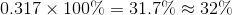# High School Physics : Understanding Heat Engines

## Example Questions

### Example Question #9 : Heat

A Carnot Cycle operates using a heat reservoir at a temperature of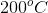and a cold reservoir at a temperature of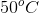. What is the operating efficiency,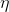, of this cycle, to the nearest percent?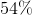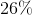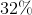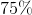Explanation:

The efficiency of a Carnot Cycle is defined by:In this calculation, temperatures are given in Kelvin. Since Celsius and Kelvin exist in a one-to-one ratio, a conversion is unnecessary unless desired. We will perform the conversion, since most classes will advise it.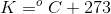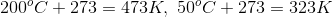Using these values, we can calculate the efficiency: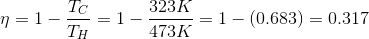Convert this value to a percentage: